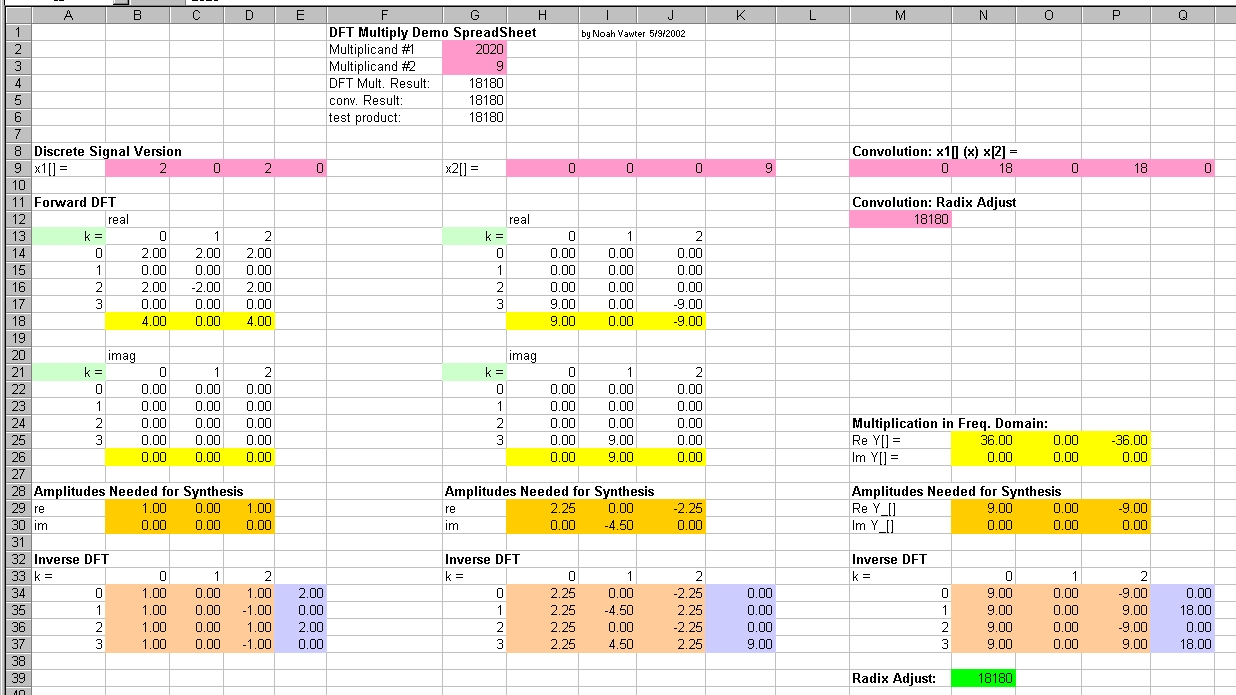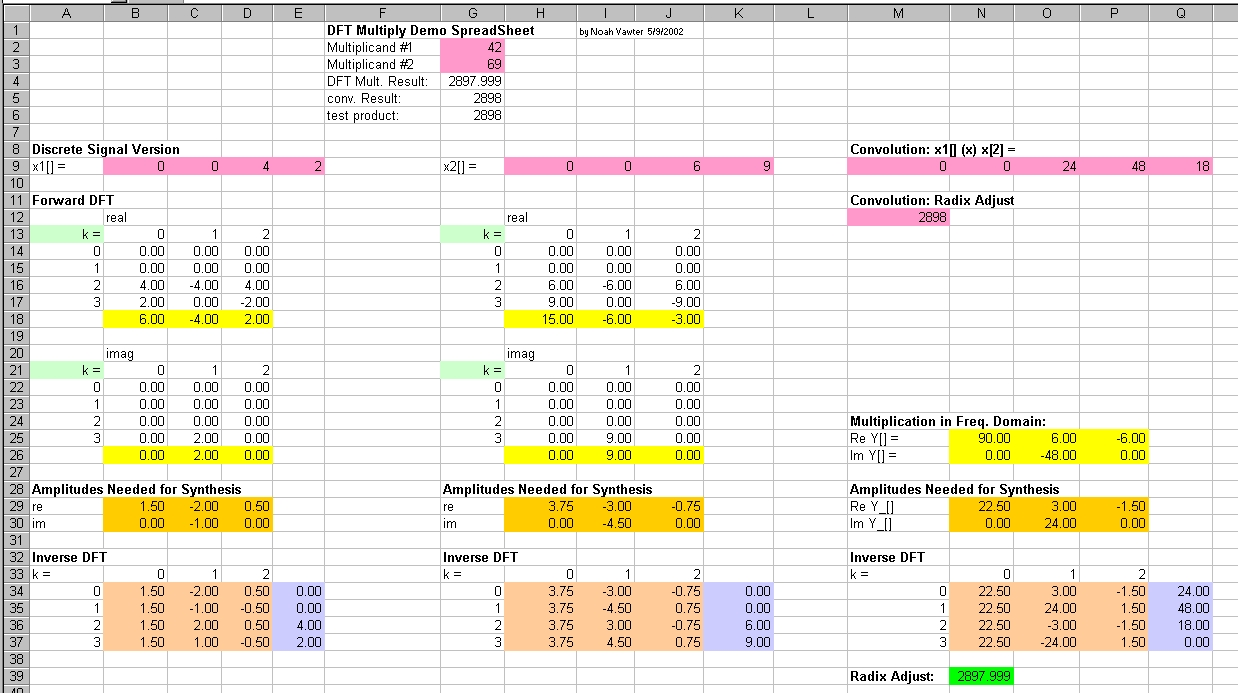## How Exactly Do FFT Multiplies Actually Work?

When I was getting interested in Mersenne prime numbers, I learned the Lucas-Lehmer test depends heavily on an FFT multiply. If you are unfamiliar with it, I'll explain: Instead of multiplying two large numbers, you take their Fourier Transforms, convolve them, then invert. At first, that sounded to me like much more trouble than actually multiplying. In fact, I was healthily skeptical. Therefore, I decided to create a dynamic environment where I could experiment and see everything taking place down to the arithmetic level!

If you are curious, you may take a look at the spreadsheet (xls format) and experiment with it. Enter your test numbers in cells G2 and G3. I highly encourage it.

## Example #1

Just in case you can't experiment with it right now, I included two example pictures.

In this first one, look at the purple cells. I am multiplying 2020 times 9 (why those numbers? you'll see). I took a DFT of each of the cells (there was no need to do an FFT to understand the multiplication aspect) and you can see those results in yellow. But, as I learned while doing this, the yellow is really the important values, you want to see look to the orange cells, the "Amplitudes needed for resynthesis.

As you can tell from imaging a signal of 2,0,2,0, there is a DC offset of 1. That is represented by the 1 in cell B29. If the FT of the signal were only 1, it would look like 1,1,1,1 however, there is another signal superimposed: 1,-1,1,-1. Of course, the period of that signal is 2, with phase of 0, and amplitude of 1, so the cell in D29 contains a 1 as well. Since there is no superposition of a signal with period 1 (corresponding to a value like 1,1,0,0), you can see that cell C29 is zero.

Finally, just to make sure my DFT routine was working, I took the inverse below it. The results are in lavender, and of course match the original value.

Over in cell N29, you can see the multiplication, and below that, the inverse which produces the correct result. The result is compared to the original in cell G7.## Example #2

In this example, I multiply a different pair of numbers to provide a further example.